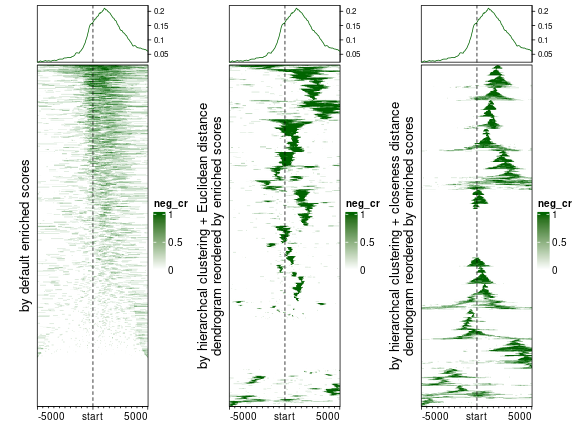# Compare row ordering methods

Author: Zuguang Gu ( z.gu@dkfz.de )

Date: 2022-04-26

library(EnrichedHeatmap)
all_genes = all_genes[unique(neg_cr\$gene)]
all_tss = promoters(all_genes, upstream = 0, downstream = 1)
mat_neg_cr = normalizeToMatrix(neg_cr, all_tss, mapping_column = "gene", w = 50, mean_mode = "w0")


The object mat_neg_cr is a normalized matrix for regions showing significant negative correlation between methylation and gene expression. The negative correlated regions (negCRs) are normalized to upstream 5kb and downstream 5kb of gene TSS with 50bp window by normalizeToMatrix() function. The value in the matrix is how much a window is covered by negCRs (values between 0 and 1).

In the normalized matrix, each row corresponds to one gene and each column corresponds to a window either on upstream of TSS or downstream of TSS. For the example of mat_neg_cr matrix, the first half columns correspond to the upstream of TSS and the last half columns correspond to downstream of TSS. Here we compare following three different methods to order rows (which correspond to genes) in the normalized matrix.

1. Rows are ordered by the enriched scores. For each row in the matrix, denote values in a certain row as $$x$$, indices 1, …, $$n_1$$ are for upstream windows, indices $$n_1+1$$, …, $$n$$ are for downstream windows and $$n_2 = n - n_1$$, the enriched score is calculated as the sum of $$x$$ weighted by distance to TSS (higher weight if the window is close to TSS).

$\sum_{i=1}^{n_1}{x_i \cdot i/n_1} + \sum_{i=n_1+1}^n{x_i \cdot (n - i + 1)/n_2}$

2. Rows are ordered by hierarchical clustering with Euclidean distance.

3. Rows are ordered by hierarchical clustering with closeness distance. For two rows in the normalized matrix, assume $$a_1, a_2, …, a_{n_1}$$ are the indices of windows for one gene which overlap with negCRs and $$b_1, b_2, … b_{n_2}$$ are the indices for the other gene which overlap with negCRs, the distance which is based on closeness of the overlapped windows in the two genes is defined as:

$d_{closeness} = \frac{\sum_{i=1}^{n_1} \sum_{j=1}^{n_2} {|a_i - b_j|} }{n_1 \cdot n_2}$

So the closeness distance is basically the average distance of all pairs of negCR windows in the two genes.

Euclidean distance between rows keeps unchanged when the matrix columns are permutated, while for closeness distance, the column order is also taken into account, which might be more proper for clustering normalized matrices because the columns correspond to relative distance to the target regions.

Following three plots show heatmaps under different row ordering methods.

EnrichedHeatmap(mat_neg_cr, name = "neg_cr", col = c("white", "darkgreen"),
top_annotation = HeatmapAnnotation(enrich = anno_enriched(gp = gpar(col = "darkgreen"))),
row_title = "by default enriched scores")

EnrichedHeatmap(mat_neg_cr, name = "neg_cr", col = c("white", "darkgreen"),
top_annotation = HeatmapAnnotation(enrich = anno_enriched(gp = gpar(col = "darkgreen"))),
cluster_rows = TRUE,
row_title = "by hierarchcal clustering + Euclidean distance\ndendrogram reordered by enriched scores")

EnrichedHeatmap(mat_neg_cr, name = "neg_cr", col = c("white", "darkgreen"),
top_annotation = HeatmapAnnotation(enrich = anno_enriched(gp = gpar(col = "darkgreen"))),
cluster_rows = TRUE, clustering_distance_rows = dist_by_closeness,
row_title = "by hierarchcal clustering + closeness distance\ndendrogram reordered by enriched scores")Generally, when the top annotation which summarises mean enrichment across genes is also added to the heatmap, ordering genes by enriched scores is not recommended because it provides redundant information as the top enriched annotation, and on the other hand, it fails to reveal spatial clusters as other two methods. Hierarchal clustering with Euclidean distance is good at clustering enrichment patterns, but since it does not take column order into account, thus, it still can be possible that two spatially close clusters are far separated in the heatmap. By using closeness distance, it clearly sorts and clusters the enrichment patterns.

The row order, clustering method, distance method can all be self-adjusted by row_order, cluster_rows, clustering_method_rows, clustering_distance_rows arguments in EnrichedHeamtap() function. For how to properly set values for these arguments, users can go to the help page of EnrichedHeatmap() or Heatmap() function.